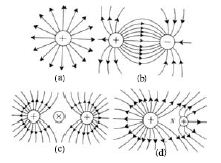# Explain Electric Lines of Force

Michel Faraday introduced electric lines of force to get an idea about electric field. If a positive charge is placed in an electric field it would experience a force. If the charge is a free one, gaining this force instead of remaining stationary it would move in a definite path. Electric line of force is the path of a free positive charge that moves in an electric field. There is no real existence of lines of force. These lines are imaginary.

The electric lines of force are used to for measuring the electric intensity and explaining its direction at a point in an electric field. The lines of force of an electric field are such that, the tangent drawn at a point to a line of force indicates the direction of electric intensity at that point. The number of lines of force passing through unit area perpendicular to the lines of force at a point in the electric field is proportional to magnitude of the electric intensity at that point. In a diagram of lines of force of an electric field, the gap between the lines indicates the magnitude of intensity of electric field. In an electric field where the lines of forces are closer magnitude of E is greater there and where the lines of forces are away magnitude of E is less there.

For different positions of charged object, the nature of the lines of force of an electric field varies. Lines of force of a few electric are described below. For the simplicity of description the conductors are taken as spherical.

• For an isolated positive charge the nature of lines of force is shown in figure (a). In this case the lines of force emerged uniformly from the surface of the conductor perpendicularly. If the charge of the body increases then the number of lines of force also increases.• The lines of force of an electric field produced by two equal and opposite charges are shown in figure (b). In this case the line of force emerges from positive charge and terminates at negative charge.
• The lines of force of an electric field produced by two equal positive charges placed nearby are shown in figure (c). In this case the lines of force go far away from each other, as a result there will be no lines of force in between them. In figure this place is indicated by ‘X’ sign. If a charge is placed at this place it will experience no force. This point is called neutral force.
• The lines of force of an electric field produced by two unequal positive charges placed nearby are shown in figure (d). In this case, the neutral point ‘N’ would not be nearer to the smaller charges.